# DC04 Image Compression Standards.pdf

29. May 2023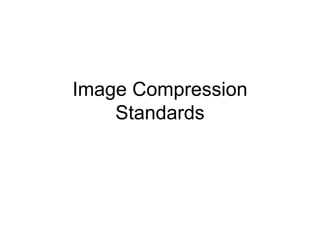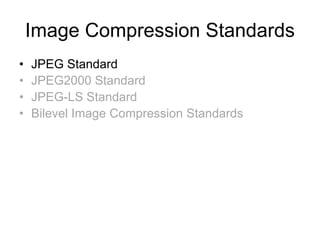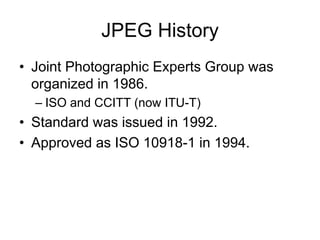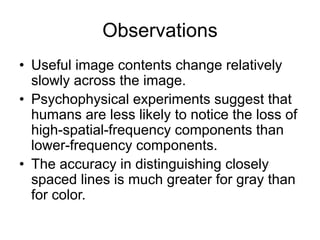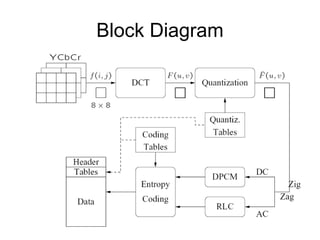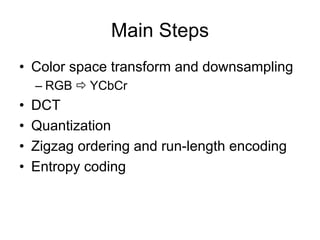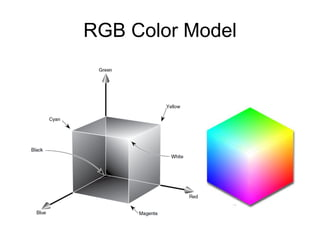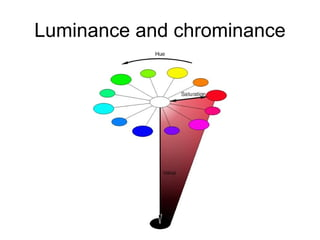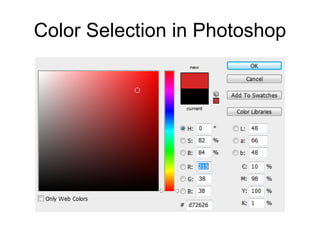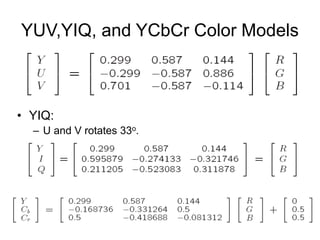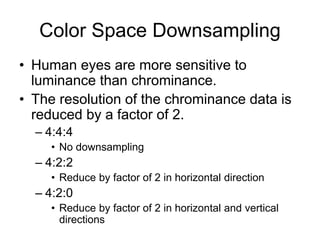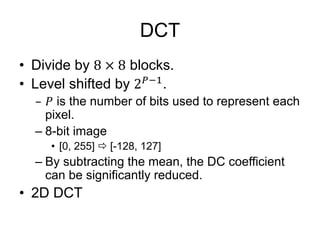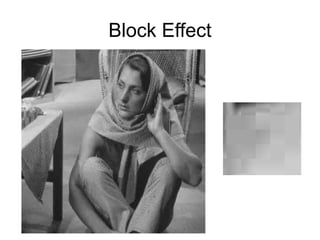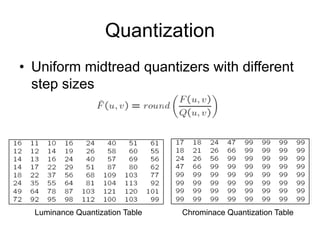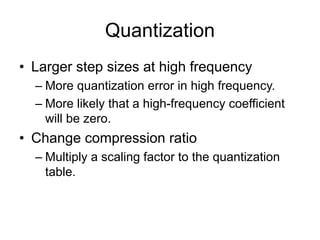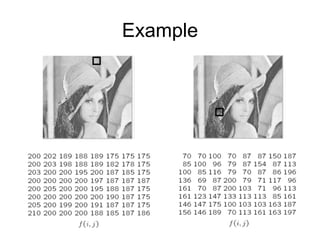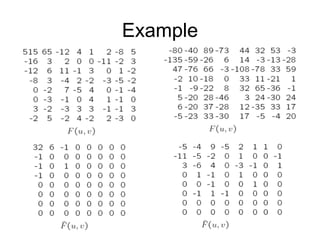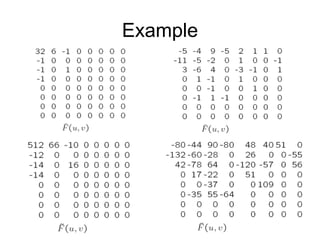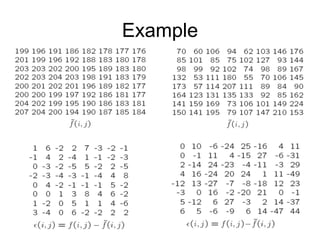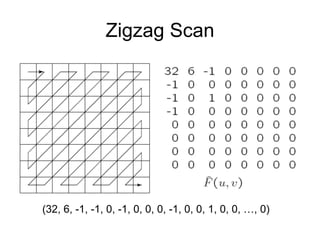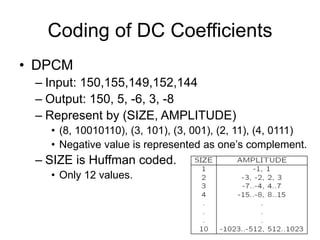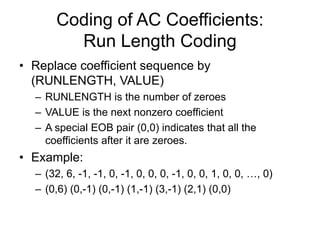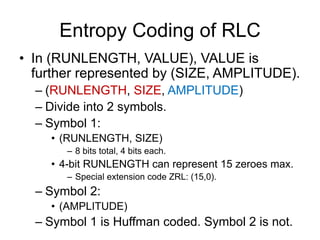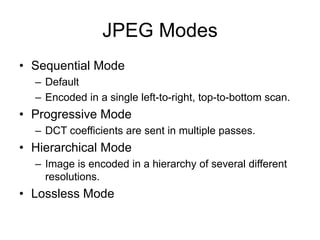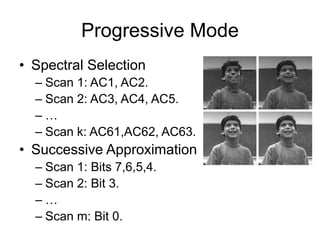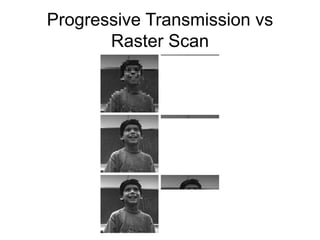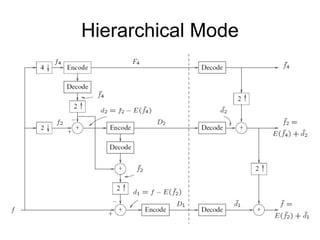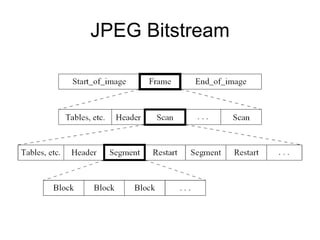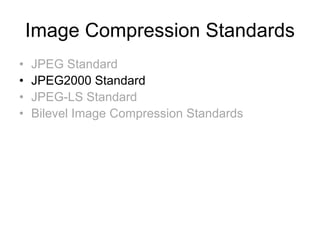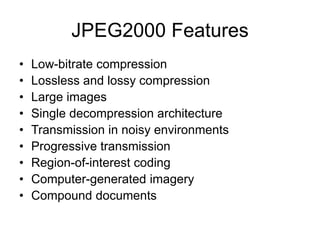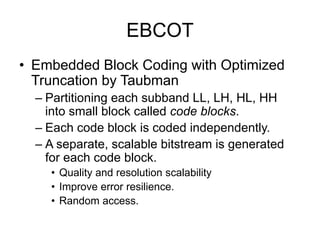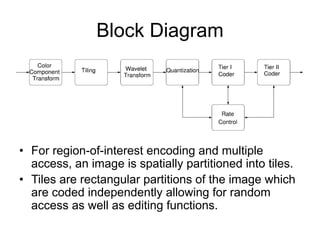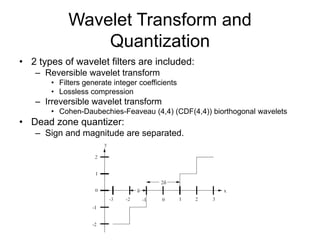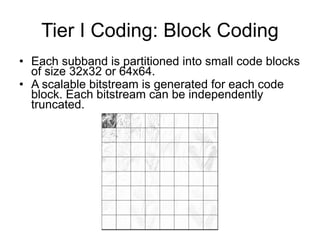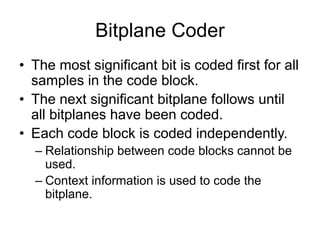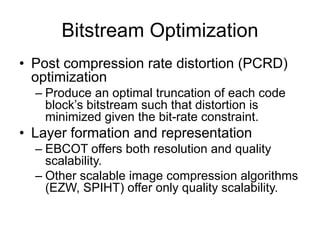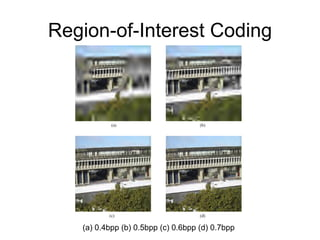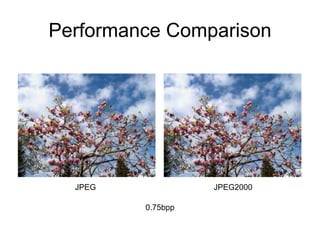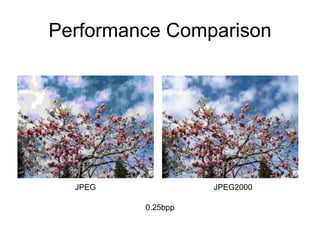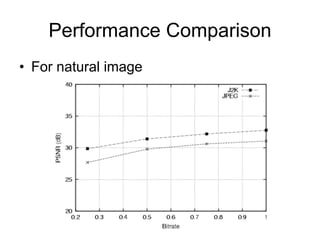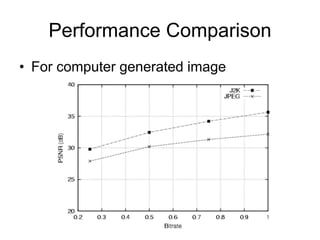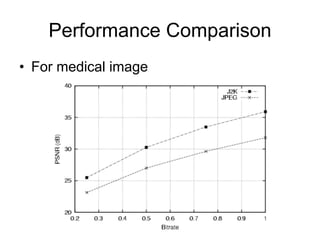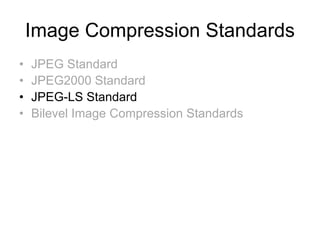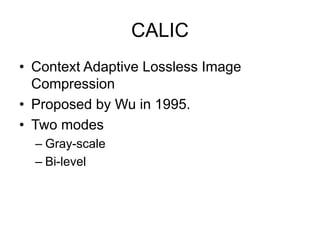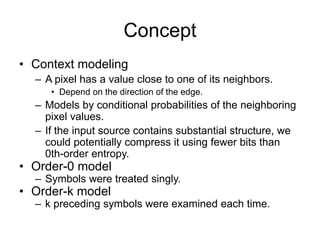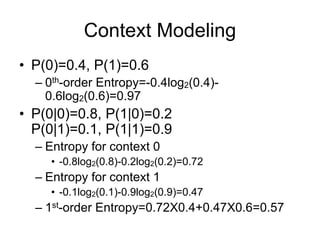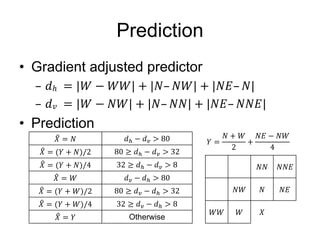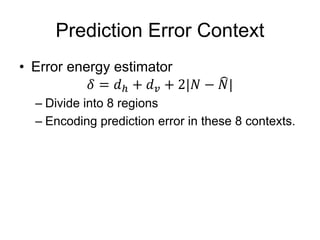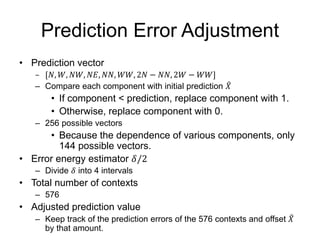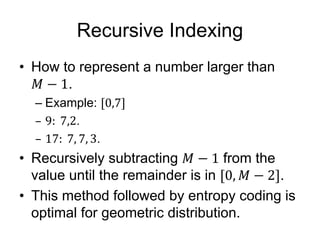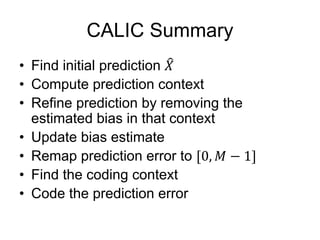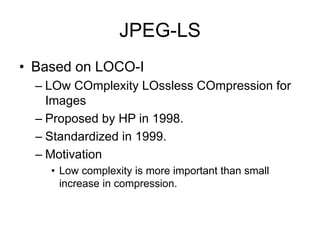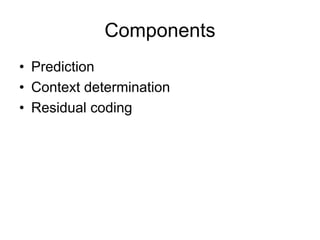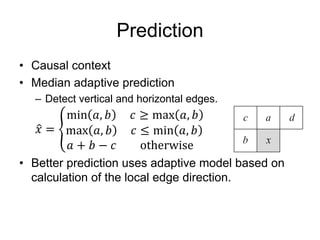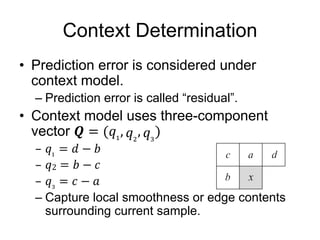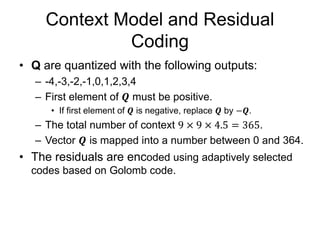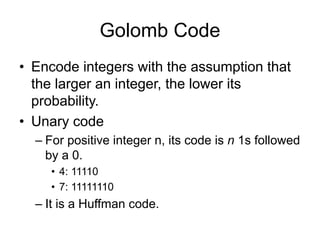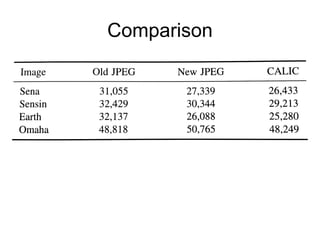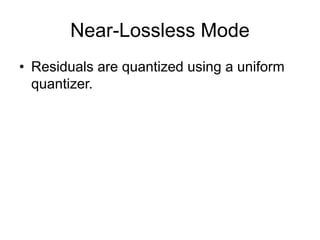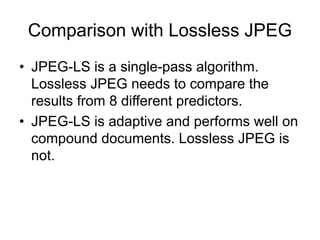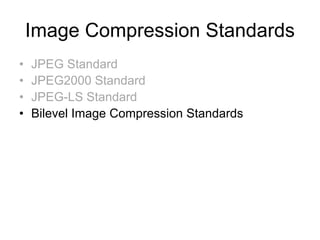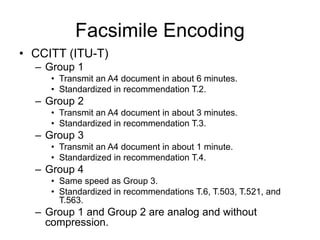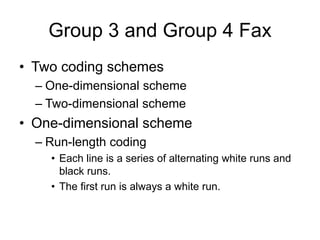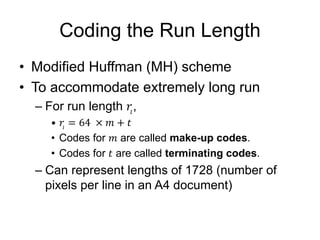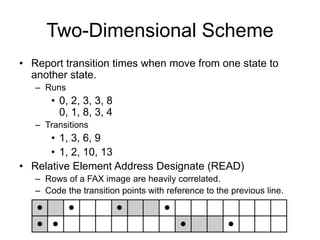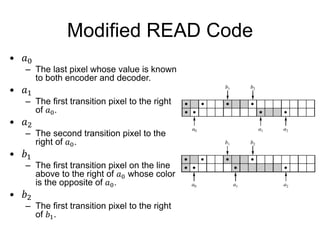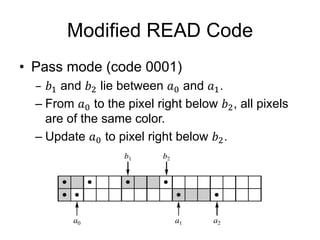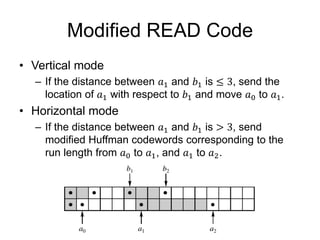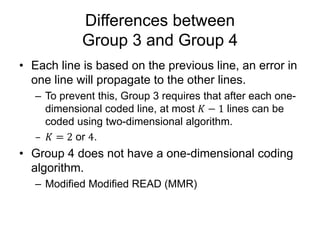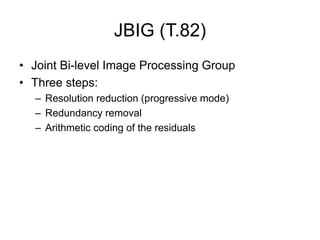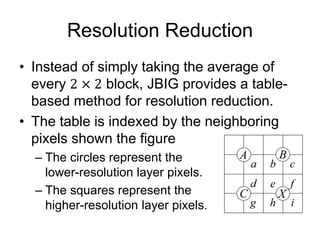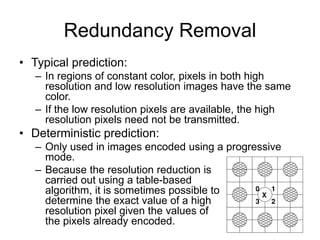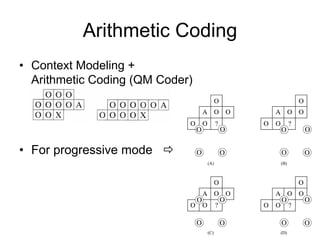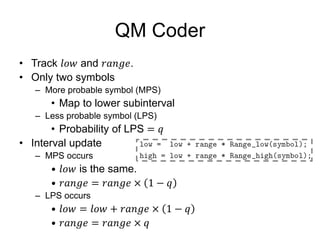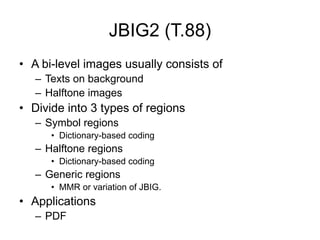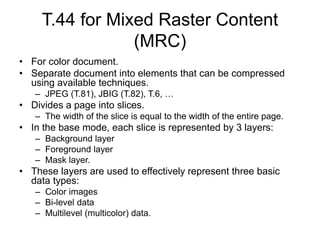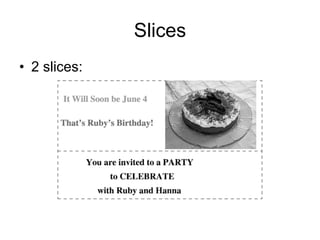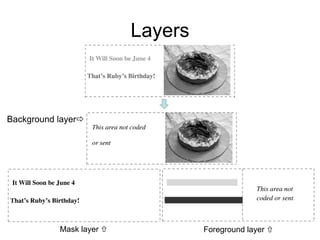1 von 78

### DC04 Image Compression Standards.pdf

• 2. Image Compression Standards • JPEG Standard • JPEG2000 Standard • JPEG-LS Standard • Bilevel Image Compression Standards
• 3. JPEG History • Joint Photographic Experts Group was organized in 1986. – ISO and CCITT (now ITU-T) • Standard was issued in 1992. • Approved as ISO 10918-1 in 1994.
• 4. Observations • Useful image contents change relatively slowly across the image. • Psychophysical experiments suggest that humans are less likely to notice the loss of high-spatial-frequency components than lower-frequency components. • The accuracy in distinguishing closely spaced lines is much greater for gray than for color.
• 6. Main Steps • Color space transform and downsampling – RGB  YCbCr • DCT • Quantization • Zigzag ordering and run-length encoding • Entropy coding
• 9. Color Selection in Photoshop
• 10. YUV,YIQ, and YCbCr Color Models • YIQ: – U and V rotates 33o.
• 11. Color Space Downsampling • Human eyes are more sensitive to luminance than chrominance. • The resolution of the chrominance data is reduced by a factor of 2. – 4:4:4 • No downsampling – 4:2:2 • Reduce by factor of 2 in horizontal direction – 4:2:0 • Reduce by factor of 2 in horizontal and vertical directions
• 12. DCT • Divide by blocks. • Level shifted by . is the number of bits used to represent each pixel. – 8-bit image • [0, 255]  [-128, 127] – By subtracting the mean, the DC coefficient can be significantly reduced. • 2D DCT
• 14. Quantization • Uniform midtread quantizers with different step sizes Luminance Quantization Table Chrominace Quantization Table
• 15. Quantization • Larger step sizes at high frequency – More quantization error in high frequency. – More likely that a high-frequency coefficient will be zero. • Change compression ratio – Multiply a scaling factor to the quantization table.
• 20. Zigzag Scan (32, 6, -1, -1, 0, -1, 0, 0, 0, -1, 0, 0, 1, 0, 0, …, 0)
• 21. Coding of DC Coefficients • DPCM – Input: 150,155,149,152,144 – Output: 150, 5, -6, 3, -8 – Represent by (SIZE, AMPLITUDE) • (8, 10010110), (3, 101), (3, 001), (2, 11), (4, 0111) • Negative value is represented as one’s complement. – SIZE is Huffman coded. • Only 12 values.
• 22. Coding of AC Coefficients: Run Length Coding • Replace coefficient sequence by (RUNLENGTH, VALUE) – RUNLENGTH is the number of zeroes – VALUE is the next nonzero coefficient – A special EOB pair (0,0) indicates that all the coefficients after it are zeroes. • Example: – (32, 6, -1, -1, 0, -1, 0, 0, 0, -1, 0, 0, 1, 0, 0, …, 0) – (0,6) (0,-1) (0,-1) (1,-1) (3,-1) (2,1) (0,0)
• 23. Entropy Coding of RLC • In (RUNLENGTH, VALUE), VALUE is further represented by (SIZE, AMPLITUDE). – (RUNLENGTH, SIZE, AMPLITUDE) – Divide into 2 symbols. – Symbol 1: • (RUNLENGTH, SIZE) – 8 bits total, 4 bits each. • 4-bit RUNLENGTH can represent 15 zeroes max. – Special extension code ZRL: (15,0). – Symbol 2: • (AMPLITUDE) – Symbol 1 is Huffman coded. Symbol 2 is not.
• 24. JPEG Modes • Sequential Mode – Default – Encoded in a single left-to-right, top-to-bottom scan. • Progressive Mode – DCT coefficients are sent in multiple passes. • Hierarchical Mode – Image is encoded in a hierarchy of several different resolutions. • Lossless Mode
• 25. Progressive Mode • Spectral Selection – Scan 1: AC1, AC2. – Scan 2: AC3, AC4, AC5. – … – Scan k: AC61,AC62, AC63. • Successive Approximation – Scan 1: Bits 7,6,5,4. – Scan 2: Bit 3. – … – Scan m: Bit 0.
• 29. Image Compression Standards • JPEG Standard • JPEG2000 Standard • JPEG-LS Standard • Bilevel Image Compression Standards
• 30. JPEG2000 Features • Low-bitrate compression • Lossless and lossy compression • Large images • Single decompression architecture • Transmission in noisy environments • Progressive transmission • Region-of-interest coding • Computer-generated imagery • Compound documents
• 31. EBCOT • Embedded Block Coding with Optimized Truncation by Taubman – Partitioning each subband LL, LH, HL, HH into small block called code blocks. – Each code block is coded independently. – A separate, scalable bitstream is generated for each code block. • Quality and resolution scalability • Improve error resilience. • Random access.
• 32. Block Diagram • For region-of-interest encoding and multiple access, an image is spatially partitioned into tiles. • Tiles are rectangular partitions of the image which are coded independently allowing for random access as well as editing functions.
• 33. Wavelet Transform and Quantization • 2 types of wavelet filters are included: – Reversible wavelet transform • Filters generate integer coefficients • Lossless compression – Irreversible wavelet transform • Cohen-Daubechies-Feaveau (4,4) (CDF(4,4)) biorthogonal wavelets • Dead zone quantizer: – Sign and magnitude are separated.
• 34. Tier I Coding: Block Coding • Each subband is partitioned into small code blocks of size 32x32 or 64x64. • A scalable bitstream is generated for each code block. Each bitstream can be independently truncated.
• 35. Bitplane Coder • The most significant bit is coded first for all samples in the code block. • The next significant bitplane follows until all bitplanes have been coded. • Each code block is coded independently. – Relationship between code blocks cannot be used. – Context information is used to code the bitplane.
• 36. Bitstream Optimization • Post compression rate distortion (PCRD) optimization – Produce an optimal truncation of each code block’s bitstream such that distortion is minimized given the bit-rate constraint. • Layer formation and representation – EBCOT offers both resolution and quality scalability. – Other scalable image compression algorithms (EZW, SPIHT) offer only quality scalability.
• 37. Region-of-Interest Coding (a) 0.4bpp (b) 0.5bpp (c) 0.6bpp (d) 0.7bpp
• 41. Performance Comparison • For computer generated image
• 43. Image Compression Standards • JPEG Standard • JPEG2000 Standard • JPEG-LS Standard • Bilevel Image Compression Standards
• 44. CALIC • Context Adaptive Lossless Image Compression • Proposed by Wu in 1995. • Two modes – Gray-scale – Bi-level
• 45. Concept • Context modeling – A pixel has a value close to one of its neighbors. • Depend on the direction of the edge. – Models by conditional probabilities of the neighboring pixel values. – If the input source contains substantial structure, we could potentially compress it using fewer bits than 0th-order entropy. • Order-0 model – Symbols were treated singly. • Order-k model – k preceding symbols were examined each time.
• 46. Context Modeling • P(0)=0.4, P(1)=0.6 – 0th-order Entropy=-0.4log2(0.4)- 0.6log2(0.6)=0.97 • P(0|0)=0.8, P(1|0)=0.2 P(0|1)=0.1, P(1|1)=0.9 – Entropy for context 0 • -0.8log2(0.8)-0.2log2(0.2)=0.72 – Entropy for context 1 • -0.1log2(0.1)-0.9log2(0.9)=0.47 – 1st-order Entropy=0.72X0.4+0.47X0.6=0.57
• 47. Prediction • Gradient adjusted predictor ℎ 𝑣 • Prediction 𝑋 𝑁 𝑑 𝑑 80 𝑋 𝑌 𝑁 /2 80 𝑑 𝑑 32 𝑋 𝑌 𝑁 /4 32 𝑑 𝑑 8 𝑋 𝑊 𝑑 𝑑 80 𝑋 𝑌 𝑊 /2 80 𝑑 𝑑 32 𝑋 𝑌 𝑊 /4 32 𝑑 𝑑 8 𝑋 𝑌 Otherwise 𝑌 𝑁 𝑊 2 𝑁𝐸 𝑁𝑊 4 𝑁𝑁 𝑁𝑁𝐸 𝑁𝑊 𝑁 𝑁𝐸 𝑊𝑊 𝑊 𝑋
• 48. Prediction Error Context • Error energy estimator – Divide into 8 regions – Encoding prediction error in these 8 contexts.
• 49. Prediction Error Adjustment • Prediction vector – 𝑁, 𝑊, 𝑁𝑊, 𝑁𝐸, 𝑁𝑁, 𝑊𝑊, 2𝑁 𝑁𝑁, 2𝑊 𝑊𝑊 – Compare each component with initial prediction 𝑋 • If component < prediction, replace component with 1. • Otherwise, replace component with 0. – 256 possible vectors • Because the dependence of various components, only 144 possible vectors. • Error energy estimator 𝛿/2 – Divide 𝛿 into 4 intervals • Total number of contexts – 576 • Adjusted prediction value – Keep track of the prediction errors of the 576 contexts and offset 𝑋 by that amount.
• 50. Recursive Indexing • How to represent a number larger than . – Example: . . • Recursively subtracting from the value until the remainder is in . • This method followed by entropy coding is optimal for geometric distribution.
• 51. CALIC Summary • Find initial prediction • Compute prediction context • Refine prediction by removing the estimated bias in that context • Update bias estimate • Remap prediction error to • Find the coding context • Code the prediction error
• 52. JPEG-LS • Based on LOCO-I – LOw COmplexity LOssless COmpression for Images – Proposed by HP in 1998. – Standardized in 1999. – Motivation • Low complexity is more important than small increase in compression.
• 53. Components • Prediction • Context determination • Residual coding
• 54. Prediction • Causal context • Median adaptive prediction – Detect vertical and horizontal edges. • Better prediction uses adaptive model based on calculation of the local edge direction.
• 55. Context Determination • Prediction error is considered under context model. – Prediction error is called “residual”. • Context model uses three-component vector 2 – Capture local smoothness or edge contents surrounding current sample.
• 56. Context Model and Residual Coding • Q are quantized with the following outputs: – -4,-3,-2,-1,0,1,2,3,4 – First element of 𝑸 must be positive. • If first element of 𝑸 is negative, replace 𝑸 by 𝑸. – The total number of context 9 9 4.5 365. – Vector 𝑸 is mapped into a number between 0 and 364. • The residuals are encoded using adaptively selected codes based on Golomb code.
• 57. Golomb Code • Encode integers with the assumption that the larger an integer, the lower its probability. • Unary code – For positive integer n, its code is n 1s followed by a 0. • 4: 11110 • 7: 11111110 – It is a Huffman code.
• 59. Near-Lossless Mode • Residuals are quantized using a uniform quantizer.
• 60. Comparison with Lossless JPEG • JPEG-LS is a single-pass algorithm. Lossless JPEG needs to compare the results from 8 different predictors. • JPEG-LS is adaptive and performs well on compound documents. Lossless JPEG is not.
• 61. Image Compression Standards • JPEG Standard • JPEG2000 Standard • JPEG-LS Standard • Bilevel Image Compression Standards
• 62. Facsimile Encoding • CCITT (ITU-T) – Group 1 • Transmit an A4 document in about 6 minutes. • Standardized in recommendation T.2. – Group 2 • Transmit an A4 document in about 3 minutes. • Standardized in recommendation T.3. – Group 3 • Transmit an A4 document in about 1 minute. • Standardized in recommendation T.4. – Group 4 • Same speed as Group 3. • Standardized in recommendations T.6, T.503, T.521, and T.563. – Group 1 and Group 2 are analog and without compression.
• 63. Group 3 and Group 4 Fax • Two coding schemes – One-dimensional scheme – Two-dimensional scheme • One-dimensional scheme – Run-length coding • Each line is a series of alternating white runs and black runs. • The first run is always a white run.
• 64. Coding the Run Length • Modified Huffman (MH) scheme • To accommodate extremely long run – For run length , • 𝑟 64 𝑚 𝑡 • Codes for 𝑚 are called make-up codes. • Codes for 𝑡 are called terminating codes. – Can represent lengths of 1728 (number of pixels per line in an A4 document)
• 65. Two-Dimensional Scheme • Report transition times when move from one state to another state. – Runs • 0, 2, 3, 3, 8 0, 1, 8, 3, 4 – Transitions • 1, 3, 6, 9 • 1, 2, 10, 13 • Relative Element Address Designate (READ) – Rows of a FAX image are heavily correlated. – Code the transition points with reference to the previous line.
• 66. Modified READ Code • 𝑎 – The last pixel whose value is known to both encoder and decoder. • 𝑎 – The first transition pixel to the right of 𝑎 . • 𝑎 – The second transition pixel to the right of 𝑎 . • 𝑏 – The first transition pixel on the line above to the right of 𝑎 whose color is the opposite of 𝑎 . • 𝑏 – The first transition pixel to the right of 𝑏 .
• 67. Modified READ Code • Pass mode (code 0001) and lie between and . – From to the pixel right below , all pixels are of the same color. – Update to pixel right below .
• 68. Modified READ Code • Vertical mode – If the distance between 𝑎 and 𝑏 is 3, send the location of 𝑎 with respect to 𝑏 and move 𝑎 to 𝑎 . • Horizontal mode – If the distance between 𝑎 and 𝑏 is 3, send modified Huffman codewords corresponding to the run length from 𝑎 to 𝑎 , and 𝑎 to 𝑎 .
• 69. Differences between Group 3 and Group 4 • Each line is based on the previous line, an error in one line will propagate to the other lines. – To prevent this, Group 3 requires that after each one- dimensional coded line, at most 𝐾 1 lines can be coded using two-dimensional algorithm. – 𝐾 2 or 4. • Group 4 does not have a one-dimensional coding algorithm. – Modified Modified READ (MMR)
• 70. JBIG (T.82) • Joint Bi-level Image Processing Group • Three steps: – Resolution reduction (progressive mode) – Redundancy removal – Arithmetic coding of the residuals
• 71. Resolution Reduction • Instead of simply taking the average of every block, JBIG provides a table- based method for resolution reduction. • The table is indexed by the neighboring pixels shown the figure – The circles represent the lower-resolution layer pixels. – The squares represent the higher-resolution layer pixels.
• 72. Redundancy Removal • Typical prediction: – In regions of constant color, pixels in both high resolution and low resolution images have the same color. – If the low resolution pixels are available, the high resolution pixels need not be transmitted. • Deterministic prediction: – Only used in images encoded using a progressive mode. – Because the resolution reduction is carried out using a table-based algorithm, it is sometimes possible to determine the exact value of a high resolution pixel given the values of the pixels already encoded.
• 73. Arithmetic Coding • Context Modeling + Arithmetic Coding (QM Coder) • For progressive mode 
• 74. QM Coder • Track 𝑙𝑜𝑤 and 𝑟𝑎𝑛𝑔𝑒. • Only two symbols – More probable symbol (MPS) • Map to lower subinterval – Less probable symbol (LPS) • Probability of LPS 𝑞 • Interval update – MPS occurs • 𝑙𝑜𝑤 is the same. • 𝑟𝑎𝑛𝑔𝑒 𝑟𝑎𝑛𝑔𝑒 1 𝑞 – LPS occurs • 𝑙𝑜𝑤 𝑙𝑜𝑤 𝑟𝑎𝑛𝑔𝑒 1 𝑞 • 𝑟𝑎𝑛𝑔𝑒 𝑟𝑎𝑛𝑔𝑒 𝑞
• 75. JBIG2 (T.88) • A bi-level images usually consists of – Texts on background – Halftone images • Divide into 3 types of regions – Symbol regions • Dictionary-based coding – Halftone regions • Dictionary-based coding – Generic regions • MMR or variation of JBIG. • Applications – PDF
• 76. T.44 for Mixed Raster Content (MRC) • For color document. • Separate document into elements that can be compressed using available techniques. – JPEG (T.81), JBIG (T.82), T.6, … • Divides a page into slices. – The width of the slice is equal to the width of the entire page. • In the base mode, each slice is represented by 3 layers: – Background layer – Foreground layer – Mask layer. • These layers are used to effectively represent three basic data types: – Color images – Bi-level data – Multilevel (multicolor) data.
• 78. Layers Background layer Mask layer  Foreground layer 